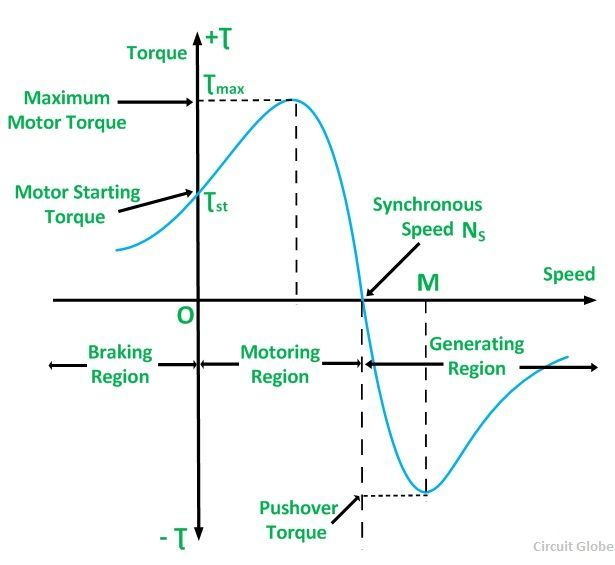# Induction Generator

The induction Generator is also known as Asynchronous Generator. An Induction Machine sometimes is used as a generator. Initially, an induction generator or machine is started as a motor. At the starting, the machine draws the lagging reactive volt-amperes from the supply mains. The speed of the machine is increased above the synchronous speed by an external prime mover. The speed is increased in the same direction as that of the rotating field produced by the stator windings.

The induction machine will operate as an induction generator and will start producing a generating torque. This generating torque is opposite to the direction of the rotation of the rotor. At this condition, the slip is negative and the induction generator starts delivering energy to the supply mains.

The torque-speed characteristics of a 3 phase induction machine for all ranges of speed is shown below:In an equivalent circuit of an induction motor, the mechanical shaft load has been replaced by a resistor of the value given below:In an induction generator, the slip (s) is negative, and therefore, the load resistance Rmech is also negative. This shows that the load resistance does not absorb the power, but starts acting as a source of power. It starts supplying the electrical energy to the supply mains to which it is connected.

The output of the induction generator depends upon the following factors given below:

• The magnitude of the negative slip.
• The speed of the rotor or how fast the motor drives above the synchronous speed in the same direction.
• Rotation of the motor when it operates as an induction motor.

It is clear from the torque-speed characteristic of the induction motor that the maximum possible induced torque occurs in the generating mode. This torque is known as Pushover Torque. If the torque becomes greater than the pushover torque, the generator will over speed.

The induction generator is not a self-excited generator. It is necessary to excite the stator with an external polyphase source to produce the rotating magnetic field. This is achieved at the rated voltage and frequency, and the machine is made to operate above the synchronous speed. Since the speed of the induction generator is different from the synchronous speed, it is known as an Asynchronous generator.

From the characteristic curve, it is seen that the operating range of the induction generator is limited to the maximum value of the pushover torque corresponding to slip at a speed OM as shown in the torque-speed characteristic curve.

### 1 thought on “Induction Generator”

1. Makarand Nandrekar

What is effect of more slip (-Ve) ( actual RPM are more than synchronous RPM) on synchronisation of Asynchronous generator?

if actual RPM changing (variation) is @ 20 RPM (for Ns of 1500) during synchronisation what is effect on synchronisation current?